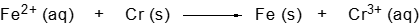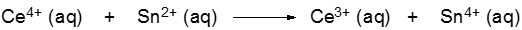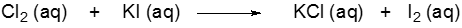## GOB Chemistry

Learn the toughest concepts covered in your GOB - General, Organic, and Biological Chemistry class with step-by-step video tutorials and practice problems.

Classification & Balancing of Chemical Reactions

# Balancing Redox Reactions (Simplified)

Balancing Redox Reactions requires a new approach that accounts for the transfer of electrons between reactants.

Balancing Redox Reactions
1
concept

## Balancing Redox Reactions (Simplified) Concept 153s
Play a video:
Balancing redox reaction requires a new approach that accounts for the transferring of electrons between reactions. Now, we're going to say here that redox reactions not only balance the atoms of elements, but also charge and a number of electrons to talk about balancing redox reactions, we take a look at half reactions. Were going to say here, balancing a redox reaction begins with identifying it's half reactions and a half reaction is either the oxidation or reduction reaction portion of a redox reaction. Now we're gonna stay here. Usually a half reaction is obtained by identifying the elements uh that are found as oxygen. Yeah, and hydrogen. So we're gonna go step by step in first identifying what half reactions are, and then later on the best way to balance and overall redox reaction.

Balancing a redox reaction begins with identifying its half reactions.

2
example

## Balancing Redox Reactions (Simplified) Example 154s
Play a video:
identify the half reactions For the following redox reaction. We have magazines, five ion reacting with quiet ion to produce magazines to ion and pouring gas. To give our two half reactions, we basically match up element ther, not oxygen and hydrogen. So manganese will be matched up with manganese. Cory will be matched up with Corey. So my first half reaction would just be the manganese five ion, giving directly the manganese to ion. And my other half reaction would be the chloride ion. Give me directly the corn gas here. Don't forget the phases that are included. So these would be Aquarius and then here this would be acquis and this would be a gas. So we have to half reactions one between the magnesium ions and one with the chlorine elements.
3
example

## Balancing Redox Reactions (Simplified) Example 23m
Play a video:
So here we're gonna balance this redox reaction here we have nickel solid plus cobalt three ion reacting to produce nickel to ion and cobalt two plus ion. So step one is we have to break the full redox reaction into two half reactions. Remember to do that. We match up elements with elements so nickel matched up with nickel ion. So that be nickel solid gives me nickel two plus acquis as one half reaction. And then cobalt with cobalt. So cobalt three ion giving me cobalt two ion. Okay, Step two We balance the overall charge by adding electrons to be more positively charged side of each half reaction. So what we're gonna say here is if we look nickel solid here has no charge that we can see if it did have a charge will be present. So it's overall charges zero. On the other side we have nickel two plus ions. So the overall charge on this side is plus two. We had electronics the more positive side so the right side and we had enough electrons so that its overall charge here will match up with the overall charge over on the other side. So I need to go from plus 2-0 in terms of overall charge. That means I need to add two electrons. Let's go to the other half reaction here. This cobalt ion is plus three. So the overall charge on this side is plus three. And then here the world charges plus two. I have to add electrons with a more positive side. So the cobalt three Ion side and I add enough electrons so that its charge overall matches the charge overall here on the lesser side. To do that, I'd have to add one electra. All right, so one half reaction has two electrons. The other one has one. Now here underneath step two we say. If the number of electrons of both reactions differ, then multiply to get the lowest common multiple. So here this is two electrons in this half reaction and this one here is one electron. So I'd have to multiply this half reaction by two. In order for this to become two electrons. Lastly we combined the half reactions and we cross out the electrons on both sides. All right, so everything is going to come down. So we're gonna have here, nickel Solid gives me Nickel two plus Aquarius Plus The two Electrons. The other half reaction, I multiplied everything by two. So that's two. C 03 plus Aquarius plus two electrons Gives me to see 02 plus acquis. So here the electrons cancel out everything else comes down. So my balance equation will be Nichols solid plus two cobalt three ions. Acquis give me one nickel two plus ion Equus Plus two. Cobalt 2 Plus A Quiz. So this year would represent my balanced redox reaction.
4
Problem

Balance the following redox reaction.5
Problem

Balance the following redox reaction.